Next: Initial Conditions in One Up: Initial Conditions on the Previous: Initial Conditions for Field

## Standing Waves: Preserving Isotropy

Equation (6.70) tells us the frequency of oscillation of the mode, but the question still remains whether we should use the plus or minus sign in the exponential. The answer is that we must use both. This fact arises from a simple property of Fourier transforms, namely that the Fourier transform of a real field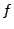must obey the symmetry(6.73)

(It doesn't matter if you are considering a complex field since you must still then set initial conditions for its real and imaginary parts, and their Fourier transforms will be constrained to obey this same symmetry relation.) We can ignore the expansion of the universe for a moment and imagine that for some modewe have chosen to use the plus sign in the exponential, i.e.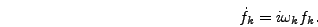(6.74)

However, since bothandare real fields it must be true that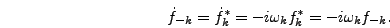(6.75)

In other words choosing the plus sign for a given momentumnecessarily means using the minus sign for the momentum. Recall that a modetranslates into a functionwith spatial dependence. So if you use the plus sign in the exponential for some positiveand the minus sign foryou have effectively initialized the two oscillatory modes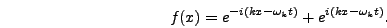(6.76)

In other words you have created a right moving wave. Likewise choosing the minus sign in the exponential for a positive value ofcorresponds to setting up a left moving wave. Of course there is no physically preferred direction on the lattice, so in reality your initial conditions should contain equal components of right and left moving fluctuations.

In practice the signs you use for the exponential time dependence of different modes has a negligible effect on the evolution once preheating begins. Even if every mode is initialized to be left-moving, the total momentum this imparts to the field is unnoticeable by the late stages of the evolution in every problem we have considered. Nonetheless it is presumably desirable to enforce Lorentz invariance, at least in an averaged sense. You could do this by randomly initializing each mode with either a plus or a minus sign. Instead, we choose to set up both left and right moving waves with equal amplitude at each value of. In other words the initial conditions correspond to standing waves. Thus the final form of the initial fluctuations is(6.77)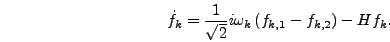(6.78)

whereand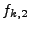are two modes with separate random phases but equal amplitudes determined by equation (6.66).

By now it may have struck you that we seem to be determining these initial conditions based on issues of convenience, symmetry, and so on. What about whatever is the physically correct form for vacuum fluctuations, as given by their quantum mechanical probability distributions? Shouldn't those distributions provide an answer to all of these questions as to the correct form of the equations? The answer is no. Although equation (6.65) gives the correct quantum distribution for the mode amplitudes, it is not correct to use this distribution and then use equation (6.72) to set the values of the field derivatives. The problem is that quantum mechanicallyand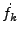are noncommuting operators and can not be simultaneously set. Although this uncertainty presents a problem in principle it is unimportant in practice. Once parametric resonance begins the occupation numbers of the modesbecome large and their quantum uncertainty becomes irrelevant. Moreover the rapid growth that occurs during this resonance effectively destroys all information about the initial values of the modes so that the final simulation results are insensitive to the details of how the initial conditions are set. In our experience runs that use the probability distribution of equation (6.65) give essentially the same results as ones that use the exact value of equation (6.68) for each mode, and likewise all qualitative results are unchanged by the use of left-moving waves, right-moving waves, or any combination of the two.Next: Initial Conditions in One Up: Initial Conditions on the Previous: Initial Conditions for Field